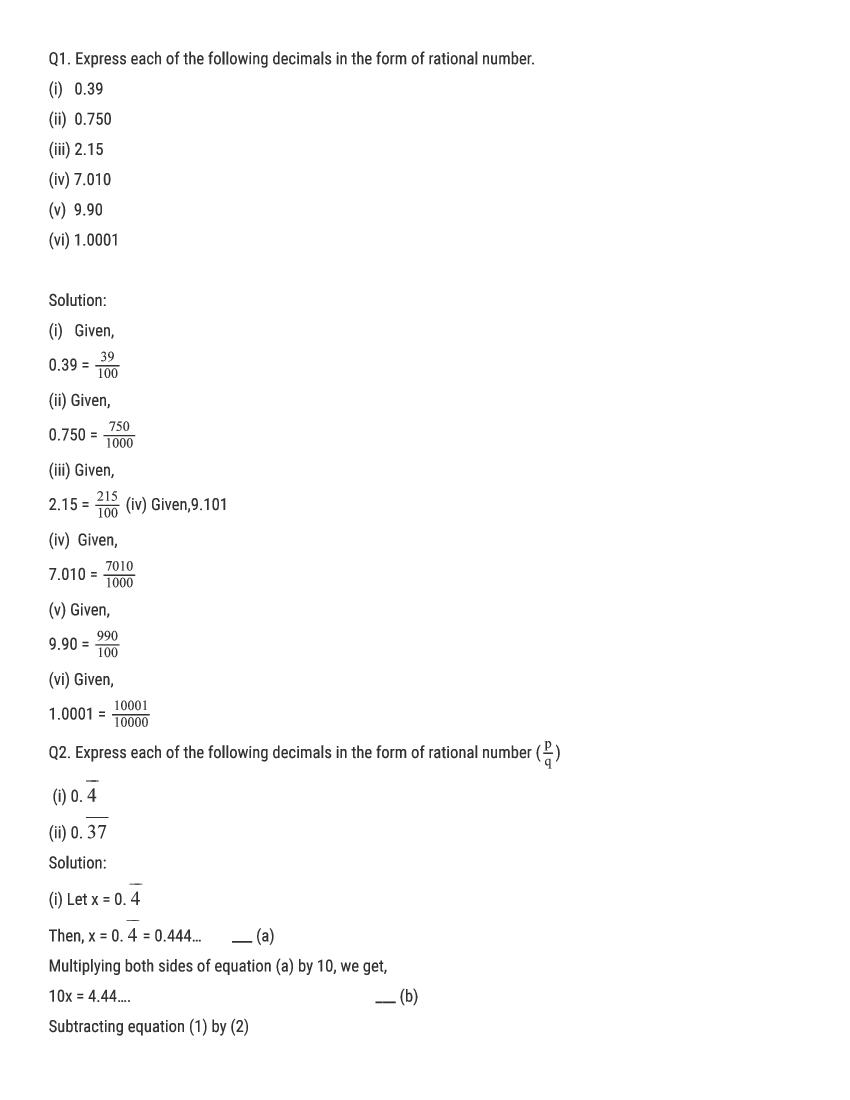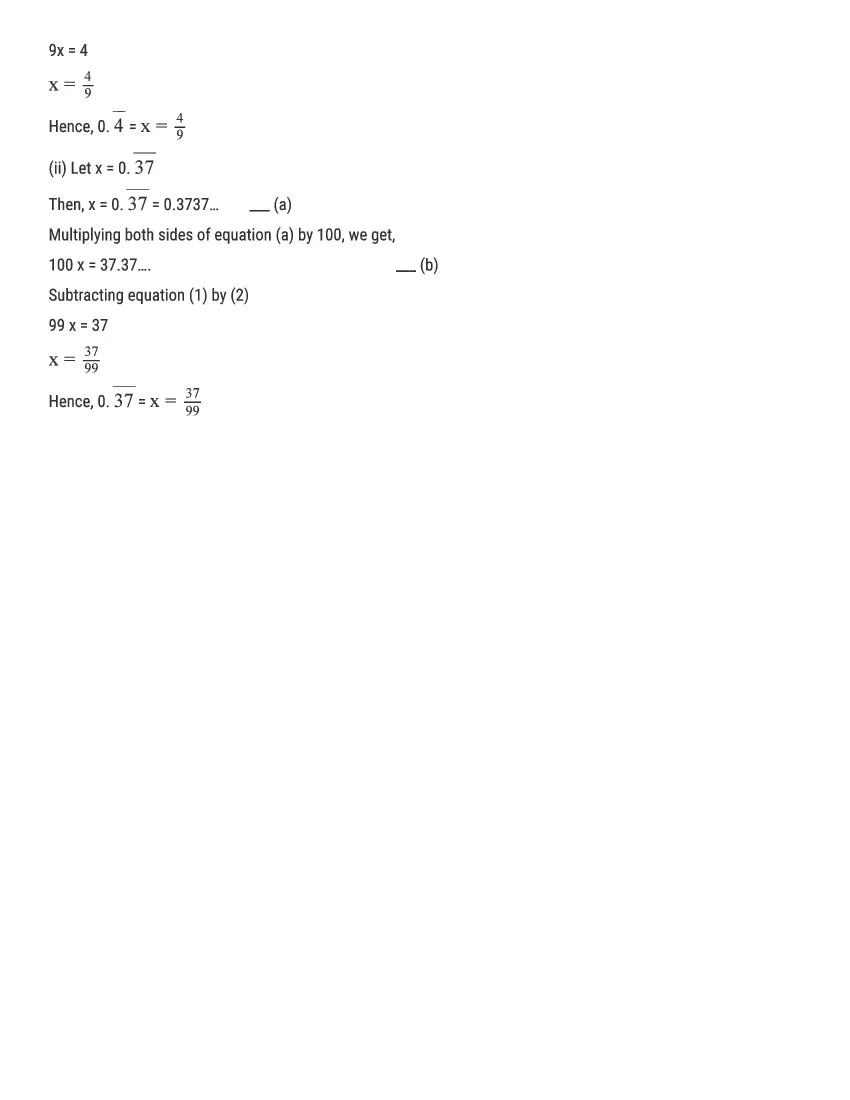# RD Sharma Solutions Class 9 Chapter 1 Number System Exercise 1.3

Here you can get free RD Sharma Solutions for Class 9 Maths Chapter 1 Number System Exercise 1.3. All RD Sharma Book Solutions are given here exercise wise for the chapter Number System. RD Sharma Solutions are helpful in the preparation of several school level, graduate and undergraduate level competitive exams. Practicing questions from RD Sharma Mathematics Solutions for Class 9 Chapter 1 Number System is proven to enhance your math skills.

 Class: Class 9th Chapter: Chapter 1 Exercise: Exercise 1.3 Name: Number System

## RD Sharma Solutions Class 9 Chapter 1 Number System Exercise 1.3

RD Sharma Class 9 Solutions Chapter 1 for Number System Exercise 1.3 are given below.

RD Sharma Solutions Class 9 Chapter 1 Number System Excercise 1.3